# How to Precisely Determine Motor Angular Position and Velocity With a Resolver

By Bonnie Baker

Contributed By Digi-Key's North American Editors

Electronic monitoring and control of mechanical systems such as industrial motors, servos, robotics, and vehicular power trains is important for greater efficiency, reliability, and safety. However, effective control requires high precision angular and velocity rotation determination, which is challenging due to the electrically noisy and rugged environment. The solution lies in a resolver supported by a precise resolver-to-digital (R/D) converter and op amps.

This article briefly discusses issues associated with achieving precise shaft measurement and control and why resolvers are a good option for many applications. It will then show how the combination of a resolver, an R/D converter such as the AD2S1210 from Analog Devices, and appropriate driver amplifier and filter circuits can create a high precision, robust position and velocity measurement and control system.

## Resolver construction

The resolver is an electromechanical device that changes mechanical motion into an analog electronic signal. It is essentially a rotary transformer with an AC voltage output that follows the angular position of the shaft. The two elements of the resolver are a single winding rotor that revolves inside the fixed stator. The resolver primary winding resides on the stator, and the secondary winding resides on the rotor (Figure 1).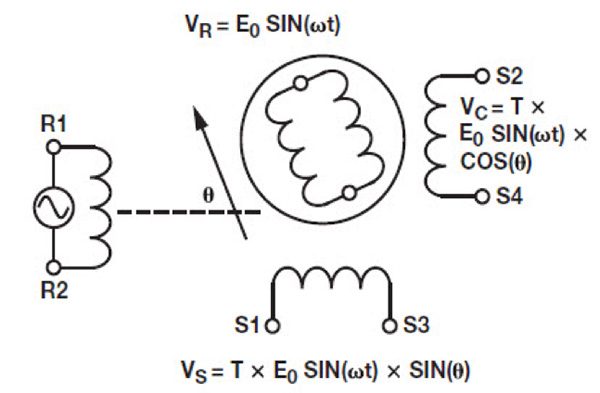Figure 1: A variable reluctance resolver has two input terminals (R1, R2), two sine output terminals (S1, S3) and two cosine output terminals (S2, S4). (Image source: Analog Devices)

Most resolver voltages are specified to be between 2 volts rms and 40 volts rms, with frequencies from 50 Hertz (Hz) to 20 kilohertz (kHz). The transformation ratio between the primary and secondary winding signal amplitude is between 0.2 volts/volt (V/V) to 1 V/V. Generally, a high performance resolver requires high input voltages, which in turn require higher power electronics to meet high output range and faster slew rate conditions. Angular accuracies range from 5 arc minutes to 0.5 arc minutes, where there are 60 arc minutes in one degree, and 60 arc seconds in one arc minute.

In Figure 1, the ac reference voltage excitation (VR = E0SIN(wt)) for the rotor winding is between R1 and R2. The induced voltage magnitude on any stator winding is proportional to the sine of angle θ, between the rotor coil axis and the stator coil axis. With a rotor ac reference voltage of E0 sinωt, the stator's terminal output voltages are:

R1 – R2 = E0 sinωt                                                                                Eq. 1

S3 – S1 = T x E0 sinωt x sin θ                                                               Eq. 2

S2 – S4 = T x E0 sinωt x sin(θ + 90°) = T x E0 sinωt x cosθ               Eq. 3

The two stator output signals are the shaft angle modulated by the sine and cosine. A graphical representation of the excitation sine signal, with a maximum amplitude at 90° and 270°, and the sine and cosine output signals have a maximum amplitude at 0° and 180° (Figure 2).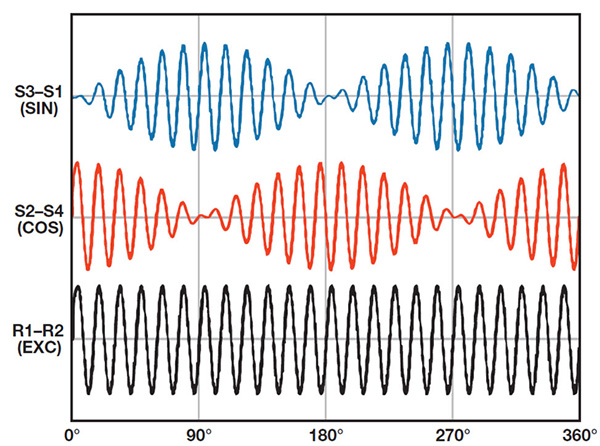Figure 2: Resolver electrical input (R1 – R2) and output signals. The two stator output signals are the shaft angle modulated by the sine and cosine. (Image source: Analog Devices)

A complete high performance R/D circuit accurately measures angular position and velocity in avionics, automotive, and critical industrial applications that require high reliability over a wide temperature range (Figure 3).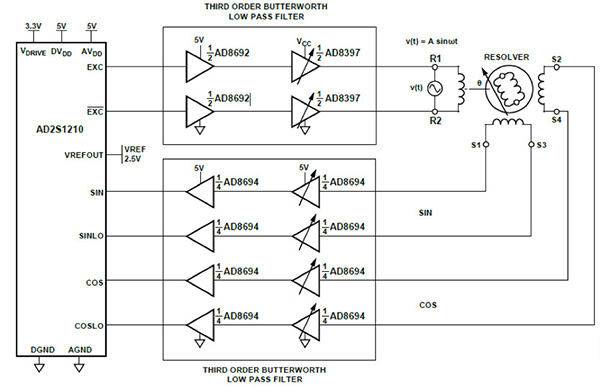Figure 3: A high performance R/D circuit, with differential output terminals (EXC:/EXC) and differential sinusoidal and cosine input terminals (SIN:SINLO, COS:COSLO). Note, EXC equals EXE in Figure 5. (Image source: Analog Devices)

In Figure 3, the R/D circuit has a resolver rotor driver circuit with two modes of operation: low power and high performance. In the low power state, the single +6 volt supply system operates while consuming less than 100 milliamps (mA). The entire system supplies 3.2 volts rms (9.2 volts p-p) to the resolver. The system in the high performance state operates with a single +12 volt supply, providing the resolver with 6.4 volts rms (18 volts p-p).

The 3rd order active filters at the R/D circuit output to resolver rotor and resolver stator outputs to the R/D circuit SIN/COS inputs, minimize the effects of the quantization noise of the system. The R/D circuit maximum tracking rate is 3125 revolutions per second (RPS) in 10-bit mode, where the resolution equals 21 arc min. In 16-bit mode, the R/D circuit maximum track rate is 156.25 RPS, giving a resolution of 19.8 arc sec.

## Signal chain design considerations

The Analog Devices AD2S1210WDSTZRL7 R/D circuit has a programmable 10, 12, 14, or 16-bit digital-to-analog converter (DAC) and 10, 12, 14, or 16-bit analog-to-digital converter (ADC), two 3rd order low-pass filters, and a resolver. The first 3rd order filter is at the output of the R/D converter into the R1 and R2 resolver rotor terminals. The second 3rd order low-pass filter collects the sinusoidal resolver stator signal at S1 and S3 and the cosine signal at S2 and S4. Generally, the system requires ample bandwidth, enough output drive capability, and an option to switch between the low power and high performance configurations.

In this circuit, the R/D circuit‘s internal DAC generates a 10, 12, 14, or 16-bit sinusoidal excitation signal with a 3.6 volt peak-to-peak, with a range from 3.2 to 4.0 volts.

At the output of the AD2S1210, there is a low-pass 3rd order filter comprising an Analog Devices AD8692ARMZ-REEL rail-to-rail op amp and an Analog Devices AD8397ARDZ-REEL7 rail-to-rail high output current amplifier.

With a +5 volt supply, the output range of the dual AD8692 low noise, CMOS op amp is 0.29 to 4.6 volts. The resistors and capacitors surrounding this amplifier implement two of the three Butterworth filter poles. The AD8397 high output current amplifier accommodates the implementation of the low-power mode, versus the high-performance mode, with a switchable gain stage and higher supply voltage capability, as well as the third pole of the low-pass filter. With a +6 volt power supply to the AD8397, the output range is 0.18 to 5.87 volts. With a +12 volt supply voltage, the output voltage range is 0.35 to 11.7 volts.

On the output side of the stator, a quad Analog Devices AD8694ARUZ-REEL low noise CMOS rail-to-rail op amp connects to the resolver’s SIN (S1 and S3) and COS (S2 and S4) pins. The AD8694, which is in the same family as the dual AD8692, has an output voltage range of 0.37 to 4.6 volts with a +5 volt power supply. The differential inputs of the AD2S1210 R/D converter (SIN, SOLO, COS, COSLO) have a peak-to-peak signal range for the resolver’s sinusoidal and cosine signals typically of 3.15 volts, with a range of 2.3 to 4.0 volts.

Ideally, in this system, the total signal chain phase shift range equals n × 180° − 44° ≤ φ ≤ n × 180° + 44°, where n is an integer.

## R/D circuit details

The signal chain design considerations include amplitude and frequency, as well as stability and phase shift, while the resolver rotor winding impedance model contains resistive and inductive elements.

The AD2S1210 R/D circuit excitation signal range is 2 kHz to 20 kHz, with increments of 250 Hz. The rotor’s applied excitation signal from the AD8397 interfaces with a non-ideal inductor and resistive component. A typical resistive and reactive component is 50 ohms (Ω) to 200 Ω, and 0 Ω to 200 Ω. Standard rotor excitation voltages can be as high as 20 volts p-p (7.1 volts rms), so it is essential to consider the resolver driver’s maximum current and maximum power consumption. To accommodate this interface, the AD8397 has a high output current (310 mA peak into 32 Ω off ±12 volt supplies), wide supply range (24 volts), low thermal resistance package (8-pin SOIC EP, θJA = 47.2°C/watt (W)), and rail-to-rail output voltage.

## Resolver excitation 3rd order filter and driver circuits

The AD2S1210 internal DAC generates the excitation output signals (EXC), which produces quantization noise and distortion (Figure 4).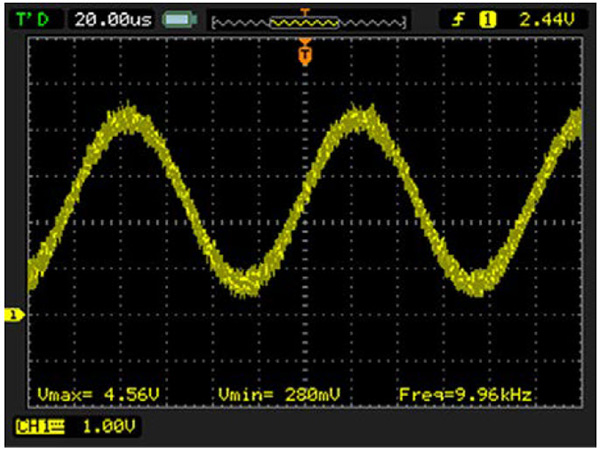Figure 4: 10 kHz output signal measured at the AD2S1210 EXC excitation output pin. (Image source: Analog Devices)

If unfiltered, the Figure 4 output noise on the AD2S1210 EXC pin will propagate through the resolver and feed back into the AD2S1210 SIN, SINLO, COS, and COSLO pins.

Also, careful attention must be paid to the gain and signal levels in the excitation circuit so that the AD8397 output driver does not saturate. The AD2S1210 output signal’s filter and power amplifier stage accommodates the rigorous requirements of the resolver’s inductive input stage (Figure 5).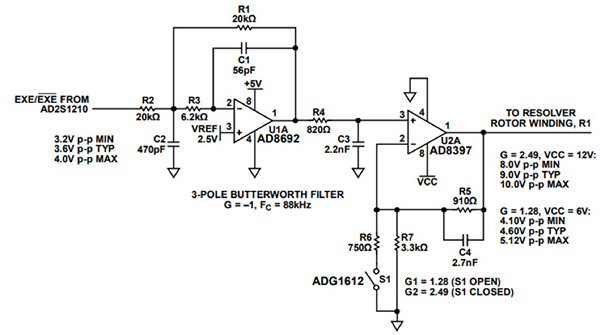Figure 5: Excitation driver and filter circuit between the R/D circuit EXC output terminals and the R1 input terminal. Note that EXE is equivalent to EXC in Figure 2. (Image source: Analog Devices)

In Figure 4, the dc gain of the AD8692 filter circuit is -1 V/V. The Analog Devices ADG1612BRUZ-REEL quad SPST switch S1 closes to create the high performance mode condition with a high VCC (≥ +12 V). With S1 closed, the gain of the AD8397 driver stage is approximately 2.5 V/V. The gain of 2.5 V/V can create a 10 volt p-p output from a 4.0 volt p-p EXE input. For the low power mode, where S1 is open, the gain equals 1.28 V/V. In this configuration, a 4.0 volt p-p EXE input produces a 5.12 volt p-p output.

The configuration of the AD8692 is a multiple feedback (MFB) third order Butterworth low pass filter. As a general rule of thumb, the amplifier gain-bandwidth product (GBWP) is at least twenty times the -3 decibel (dB) active filter’s cutoff frequency. The cutoff frequency is 88 kHz in Figure 5, and the AD8692's GBWP is 10 MHz, which is 113 times the cutoff frequency. Typically, this circuit’s phase shift is 180° ± 15°. In the circuit of Figure 4, the -3 dB cutoff frequency of the filter is 88 kHz; the phase shift is -13° at 10 kHz.

The dual AD8692 op amp serves as a 3rd order active Butterworth filter to reduce the drive signal noise (Figure 6).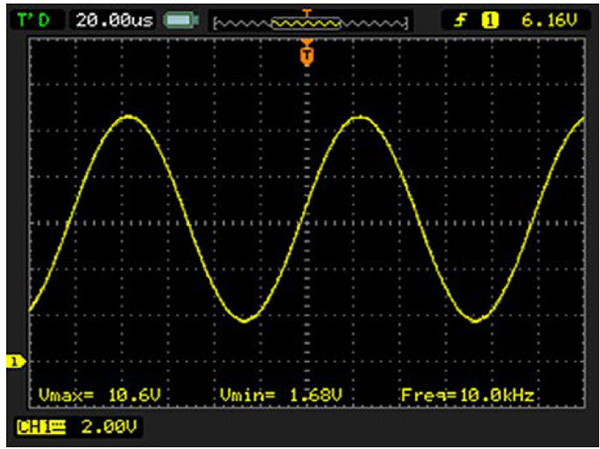Figure 6: After the R/D converter output signal travels through the excitation driver and filter, the noise riding on the signal is significantly reduced and ready for the resolver input at R1. (Image source: Analog Devices)

The data in Figure 6 demonstrates a significant reduction in the AD2S1210’s internal DAC quantization noise.

Similarly, the SIN (S1 and S3) and COS (S2 and S4) receiver circuits use two quad AD8694 op amps as an active noise filter.  The total phase shift between the AD2S1210 EXC pin (CH1 yellow) to the SIN input pin (CH2 blue) is approximately 40°, which is below the maximum design value of 44° (Figure 7).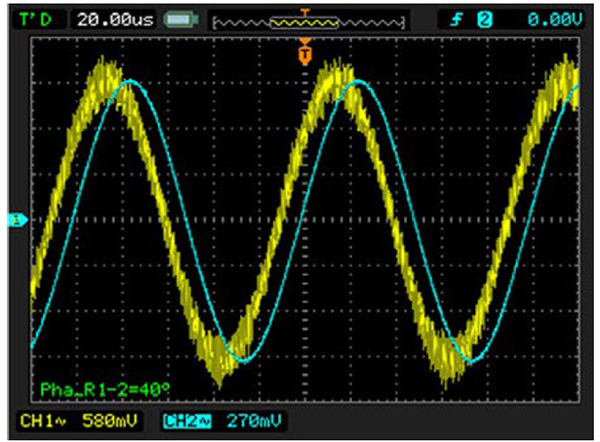Figure 7: There is a signal phase shift caused by the analog driver and filter into the resolver input, the resolver, and the analog filter back to the R/D converter. The scope screen capture illustrates the phase shift between the AD2S1210 EXC and SIN pins. (Image source: Analog Devices)

## System performance

The evaluation circuit for this article uses the Analog Devices EVAL-CN0276-SDPZ circuit board and the Analog Devices EVAL-SDP-CB1Z system platform controller board (Figure 8).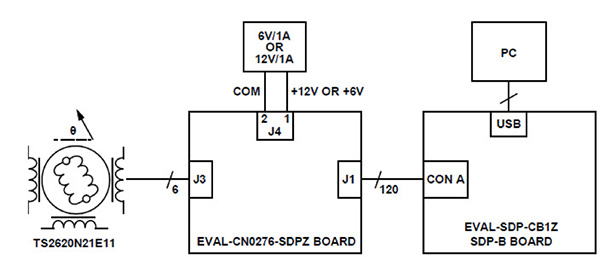Figure 8: Functional diagram of test setup corresponding to Figures 4, 6, 7, 10, and 11. (Image source: Analog Devices)

In Figure 8, 120-pin mating connectors between the two boards allow a quick setup and circuit performance evaluation.

The EVAL-CN0276-SDPZ contains the complete circuit, and the EVAL-SDP-CB1Z (SDP-B), in conjunction with the CN-0276 Evaluation Software, exchanges the data from the EVAL-CN0276-SDPZ (Figure 9).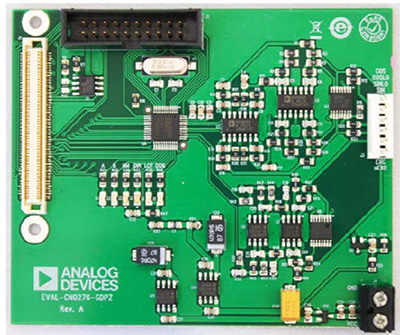Figure 9: The EVAL-CN0276-SDPZ pc board contains the complete circuit for the R/D converter. (Image source: Analog Devices)

With the overall system noise measurement, a fixed position to the resolver, a Tamagawa TS2620N21E11, generates an output code histogram. The AD2S1210’s output histogram of codes for the 10-bit and 16-bit angular accuracy modes display the combination of the transmitting DAC and receiving ADC (Figures 10 and 11). In this article, the TS2620N21E11 resolver has a 0° phase shift and a transformation ratio of 0.5. The resolver’s sinusoidal (SIN) and cosine (COS) output loads are equal and are at least twenty times the resolver’s output impedance.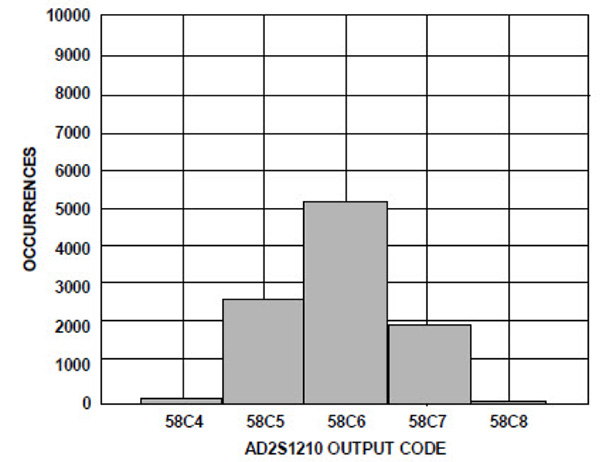Figure 10: EXE transmitting in a 10-bit angular accuracy mode, SIN/COS receiving with 16-bit ADC resolution. (Image source: Analog Devices)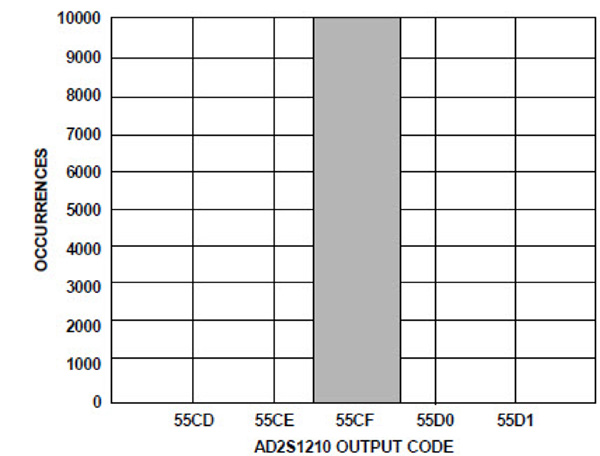Figure 11: EXC transmitting 16-bit angular accuracy mode, SIN/COS receiving with 16-bit ADC resolution. (Image source: Analog Devices)

In Figure 10 and Figure 11, VCC equals 12 volts, which places the full 16 bits of the R/D converter in its high performance mode.

## Conclusion

The combination of resolvers and an R/D converter such as the AD2S1210 from Analog Devices creates a high precision, robust position and velocity control system for potentially hostile environmentally motor control applications.

To deliver the best overall performance, the AD8694 and AD8397 combine to create buffer/filter circuits that amplify the excitation signals and provide the appropriate drive to the resolver, as well as filtering and feeding back of secondary signals. With the AD2S1210’s variable resolution, reference generation, and on-chip diagnostics, the R/D converter provides an ideal solution for resolver applications.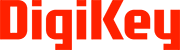Disclaimer: The opinions, beliefs, and viewpoints expressed by the various authors and/or forum participants on this website do not necessarily reflect the opinions, beliefs, and viewpoints of Digi-Key Electronics or official policies of Digi-Key Electronics.

### About this authorBonnie Baker

Bonnie Baker is a contributing author at Digi-Key Electronics. Burr-Brown, Microchip and Texas Instruments facilitated her involvement in analog design and analog systems for the last 30+ years. Bonnie holds a Masters of Science in Electrical Engineering from the University of Arizona (Tucson, AZ) and a bachelor’s degree in music education from Northern Arizona University (Flagstaff, AZ). In addition to her analog design fascination, Bonnie has a drive to share her knowledge and experience through the authorship of over 450 articles, design notes, and application notes.

### About this publisher

Digi-Key's North American Editors